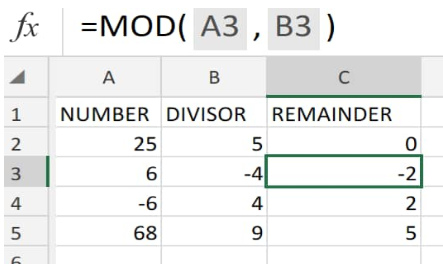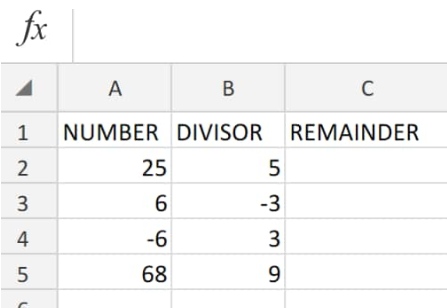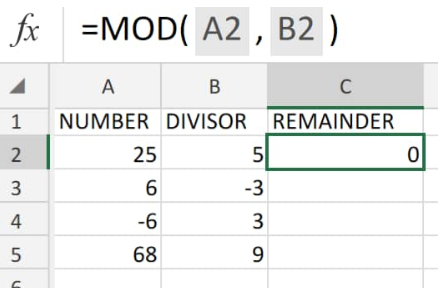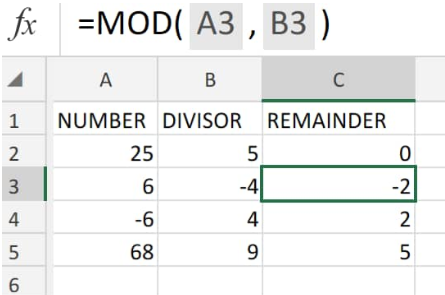Get instant live expert help with Excel or Google Sheets“My Excelchat expert helped me in less than 20 minutes, saving me what would have been 5 hours of work!”

#### Post your problem and you’ll get expert help in seconds.

Your message must be at least 40 characters
Our professional experts are available now. Your privacy is guaranteed.

# Excel Mod function – Excelchat

We can use the Mod Function Excel to calculate the remainder after numbers have been divided by a divisor.

The resulting MOD remainder in Excel and the divisor should both carry the same sign.Figure 1. of Mod Function in Excel

The MOD Excel function is an in-built function which is categorized under Math/Trig Functions.

It can be utilized as an Excel worksheet (WS) function. As a WS function, we can enter the modulo Excel operation syntax as a component part of a cell formula in our worksheet.

## Generic Formula

`=MOD (number, divisor)`

• Number = The numeric value for which we want to determine the remainder
• Divisor = The number which we want to use as the number divider.

## How to use the mod function Excel

We will now demonstrate how to use the mod formula in Excel with the following simple steps;

1. We start by arranging our number values in the appropriate worksheet columns.Figure 2. of Mod Data in Excel

The number values which we want mod Excel to return their remainder are in column A of our worksheet example, while the dividing values are in column B.

Be sure to allocate empty cells for mod in Excel to return the remainder values (column C).

1. The mod formula we will enter into cell C2 above, to determine the remainder Excel is as follows;
`=MOD(A2,B2)`Figure 3. of Mod Function in Excel

The mod formula in cell C2 automatically returns 0 as our remainder value for the number in cell A2 (25).

1. Modify and copy the Excel mod function in C2 down into the other cells in the “Remainder” column of our worksheet example; this is done to calculate the remainder Excel value for the other numbers in column A:Figure 4. of Mod Function in Excel

## Note

• We can utilize Excel MOD when extracting time values from a date.
• MOD formula in Excel is mostly used in formulas dealing with “nth” values
• The MOD remainder function Excel will return an error value – #DIV/0! – if our divisor is zero
• MOD formula will always return a remainder result that has the same (+/-) sign as its divisor.